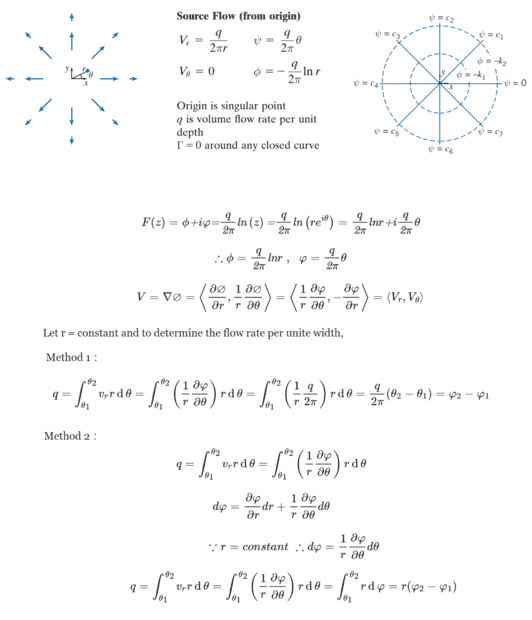# Question about flow rate in the source flow

tracker890 Source h
Homework Statement:
Determine flow rate per unite width in the source flow
Relevant Equations:
flow rate equation
ref.Flowrate Between Streamlines
(Thank you for your time and consideration.)Last edited:

Homework Helper
Gold Member
In the third line from the end where you have an equation for ##d\varphi##, the last term should not have a factor of ##\frac 1 r##. Note how the factor of ##\frac 1 r## makes the term have the wrong dimensions.

tracker890 Source h
In the third line from the end where you have an equation for ##d\varphi ##, the last term should not have a factor of ##\frac 1 r##. Note how the factor of ##\frac 1 r## makes the term have the wrong dimensions.
But about the gradent in cylindrical coordinates is ##\nabla\mathrm\psi=\frac{\partial\mathrm\psi}{\partial r}{\textbf{e}}_r+\frac1r\frac{\partial\mathrm\psi}{\partial\mathrm\theta}{\textbf{e}}_\theta+\frac{\partial\mathrm\psi}{\partial z}{\textbf{e}}_z##, so ##d\mathrm\psi=\frac{\partial\mathrm\psi}{\partial r}dr+\frac1r\frac{\partial\mathrm\psi}{\partial\mathrm\theta}d\theta+\frac{\partial\mathrm\psi}{\partial z}dz##.
Is the above thinking wrong?

Homework Helper
Gold Member
2022 Award
But about the gradent in cylindrical coordinates is ##\nabla\mathrm\psi=\frac{\partial\mathrm\psi}{\partial r}{\textbf{e}}_r+\frac1r\frac{\partial\mathrm\psi}{\partial\mathrm\theta}{\textbf{e}}_\theta+\frac{\partial\mathrm\psi}{\partial z}{\textbf{e}}_z##, so ##d\mathrm\psi=\frac{\partial\mathrm\psi}{\partial r}dr+\frac1r\frac{\partial\mathrm\psi}{\partial\mathrm\theta}d\theta+\frac{\partial\mathrm\psi}{\partial z}dz##.
Is the above thinking wrong?
Isn't ##{\textbf{e}}_\theta=rd\theta##?

tracker890 Source h
Isn't ##{\textbf{e}}_\theta=rd\theta##?
Different：
##{\textbf{e}}_\theta## is vector
##rd\theta## is scalar

Homework Helper
Gold Member
2022 Award
Different：
##{\textbf{e}}_\theta## is vector
##rd\theta## is scalar
Yes, I forgot to make the dθ bold. But the point is you need a factor r.

Homework Helper
Gold Member
2022 Award
$$d\psi=\frac{\partial\psi}{\partial r}dr+\frac{\partial\psi}{\partial\theta}d\theta$$
reference as follows：
he Gradient in Polar Coordinates and other Orthogonal Coordinate Systems
Yes, for the case of constant z; so?
Compare that with what you wrote in post #3. You have a 1/r factor on the second term which should not be there. It appears in your version because you wrongly replaced eθ with dθ instead of with rdθ. Check the dimensionality of each.

Homework Helper
Gold Member
But about the gradent in cylindrical coordinates is ##\nabla\mathrm\psi=\frac{\partial\mathrm\psi}{\partial r}{\textbf{e}}_r+\frac1r\frac{\partial\mathrm\psi}{\partial\mathrm\theta}{\textbf{e}}_\theta+\frac{\partial\mathrm\psi}{\partial z}{\textbf{e}}_z##, so ##d\mathrm\psi=\frac{\partial\mathrm\psi}{\partial r}dr+\frac1r\frac{\partial\mathrm\psi}{\partial\mathrm\theta}d\theta+\frac{\partial\mathrm\psi}{\partial z}dz##.
Is the above thinking wrong?
-----------------
##\nabla\mathrm\psi=\frac{\partial\mathrm\psi}{\partial r}{\textbf{e}}_r+\frac1r\frac{\partial\mathrm\psi}{\partial\mathrm\theta}{\textbf{e}}_\theta+\frac{\partial\mathrm\psi}{\partial z}{\textbf{e}}_z##

This equation is correct. It expresses the gradient of ##\psi##. The gradient of ##\psi## is a vector quantity. The dimensions of the gradient of ##\psi## are the dimensions of ##\psi## divided by distance. You can check that each term on the right of the equation has these dimensions.
------------------

##d\mathrm\psi=\frac{\partial\mathrm\psi}{\partial r}dr+\frac1r\frac{\partial\mathrm\psi}{\partial\mathrm\theta}d\theta+\frac{\partial\mathrm\psi}{\partial z}dz##

This equation is not correct. ##d \psi## is the differential of psi. This is a scalar quantity that represents a small change in ##\psi## when the variables ##r, \theta## and ##z## are varied by ##dr##, ##d\theta##, and ##dz## respectively. The dimensions of ##d \psi## are the same as the dimensions of ##\psi##. Each term on the right side of the equation should have dimensions of ##\psi##. But the second term on the right side of your equation has dimensions of ##\psi## divided by distance (##r##).

For an arbitrary function ##f## of three variables ##u, v, w##, the differential of ##f(u, v, w)## is $$df = \frac{\partial f}{\partial u} du + \frac{\partial f}{\partial v}dv + \frac{\partial f}{\partial w}dw$$ So ##d \psi## is $$d\mathrm\psi=\frac{\partial\mathrm\psi}{\partial r}dr+\frac{\partial\mathrm\psi}{\partial\mathrm\theta}d\theta+\frac{\partial\mathrm\psi}{\partial z}dz$$

tracker890 Source h
-----------------
##\nabla\mathrm\psi=\frac{\partial\mathrm\psi}{\partial r}{\textbf{e}}_r+\frac1r\frac{\partial\mathrm\psi}{\partial\mathrm\theta}{\textbf{e}}_\theta+\frac{\partial\mathrm\psi}{\partial z}{\textbf{e}}_z##

This equation is correct. It expresses the gradient of ##\psi##. The gradient of ##\psi## is a vector quantity. The dimensions of the gradient of ##\psi## are the dimensions of ##\psi## divided by distance. You can check that each term on the right of the equation has these dimensions.
------------------

##d\mathrm\psi=\frac{\partial\mathrm\psi}{\partial r}dr+\frac1r\frac{\partial\mathrm\psi}{\partial\mathrm\theta}d\theta+\frac{\partial\mathrm\psi}{\partial z}dz##

This equation is not correct. ##d \psi## is the differential of psi. This is a scalar quantity that represents a small change in ##\psi## when the variables ##r, \theta## and ##z## are varied by ##dr##, ##d\theta##, and ##dz## respectively. The dimensions of ##d \psi## are the same as the dimensions of ##\psi##. Each term on the right side of the equation should have dimensions of ##\psi##. But the second term on the right side of your equation has dimensions of ##\psi## divided by distance (##r##).

For an arbitrary function ##f## of three variables ##u, v, w##, the differential of ##f(u, v, w)## is $$df = \frac{\partial f}{\partial u} du + \frac{\partial f}{\partial v}dv + \frac{\partial f}{\partial w}dw$$ So ##d \psi## is $$d\mathrm\psi=\frac{\partial\mathrm\psi}{\partial r}dr+\frac{\partial\mathrm\psi}{\partial\mathrm\theta}d\theta+\frac{\partial\mathrm\psi}{\partial z}dz$$
$$d\psi=\frac{\partial\psi}{\partial r}dr+\frac{\partial\psi}{\partial\theta}d\theta+\frac{\partial\psi}{\partial z}dz$$
$$\because r\;and\;z=constant\;\;\;\therefore d\psi=\frac{\partial\psi}{\partial\theta}d\theta$$
$$d\psi=\frac{\partial\psi}{\partial r}dr+\frac{\partial\psi}{\partial\theta}d\theta+\frac{\partial\psi}{\partial z}dz$$
$$\because r\;and\;z=constant\;\;\;\therefore d\psi=\frac{\partial\psi}{\partial\theta}d\theta$$
•